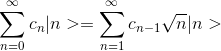# Eigenvector of raising operator

## Homework Statement

show that the raising operator at has no right eigenvectors

## Homework Equations

We know at|n> = √(n+1)|n+1>

## The Attempt at a Solution

we define a vector |Ψ> = ∑cn|n> (for n=0 to ∞)

at|Ψ>=at∑cn|n>=∑cn(√n+1)|n+1>

But further I give up!PeroK
Homework Helper
Gold Member
2021 Award

## Homework Statement

show that the raising operator at has no right eigenvectors

## Homework Equations

We know at|n> = √(n+1)|n+1>

## The Attempt at a Solution

we define a vector |Ψ> = ∑cn|n> (for n=0 to ∞)

at|Ψ>=at∑cn|n>=∑cn(√n+1)|n+1>

But further I give up!You have to try. It's not that hard.

•Pouyan
You have to try. It's not that hard.
Well I do see this :

at|Ψ>=at∑cn|n>=∑cn(√n+1)|n+1>
In a solution i do see:Latex code : \sum_{n=0}^{\infty}c_{n}|n>=\sum_{n=1}^{\infty}c_{n-1}\sqrt{n}|n>
I know how to iterate a sum serie but why shall we say so ?!

and the only possible value is c_n=0

#### Attachments

PeroK
Homework Helper
Gold Member
2021 Award
Well I do see this :

at|Ψ>=at∑cn|n>=∑cn(√n+1)|n+1>
In a solution i do see:

View attachment 230995

Latex code : \sum_{n=0}^{\infty}c_{n}|n>=\sum_{n=1}^{\infty}c_{n-1}\sqrt{n}|n>
I know how to iterate a sum serie but why shall we say so ?!

and the only possible value is c_n=0

Two things.

1) You should be writing down what it means for ##a^t## to have an eigenvector:

##a^t|\Psi \rangle = \lambda |\Psi \rangle##

2) Sometimes you can see things better using the ##\sum## notation. And sometimes you can see things better with the terms written out. If you are stuck, you should always try both ways. In this case, for example, let:

##|\Psi \rangle = c_0 |0 \rangle + c_1 |1 \rangle + c_2 |2 \rangle \dots ##

You are right that you can show that ##c_n = 0##. Can you show this fully? Also, what about the case where ##\lambda = 0## as a possible eigenvalue?

•Pouyan
Two things.

1) You should be writing down what it means for ##a^t## to have an eigenvector:

##a^t|\Psi \rangle = \lambda |\Psi \rangle##

2) Sometimes you can see things better using the ##\sum## notation. And sometimes you can see things better with the terms written out. If you are stuck, you should always try both ways. In this case, for example, let:

##|\Psi \rangle = c_0 |0 \rangle + c_1 |1 \rangle + c_2 |2 \rangle \dots ##

You are right that you can show that ##c_n = 0##. Can you show this fully? Also, what about the case where ##\lambda = 0## as a possible eigenvalue?
this is my problem, I dont get it
How can I showis right if and only if c_n = 0.....?
I know the only solution for all n must be c_n=0

#### Attachments

PeroK
Homework Helper
Gold Member
2021 Award
this is my problem, I dont get it
How can I show View attachment 231005

is right if and only if c_n = 0.....?
I know the only solution for all n must be c_n=0

You haven't followed either of my suggestions. You must at least write down what it means to have an eigenvector. Maths doesn't solve itself. You need to start working with the equations.

Anyway, the rules of PF are clear: you must start to show some of your work.

vela
Staff Emeritus
Homework Helper
In a solution i do see:
$$\sum_{n=0}^{\infty}c_{n}|n\rangle=\sum_{n=1}^{\infty}c_{n-1}\sqrt{n}|n\rangle$$
You might be getting confused because that's wrong. What it should say is
$$\hat a^\dagger \sum_{n=0}^{\infty}c_{n}|n\rangle=\sum_{n=0}^{\infty}c_{n}\sqrt{n+1}|n+1\rangle = \sum_{n=1}^{\infty}c_{n-1}\sqrt{n}|n\rangle.$$ From this alone, you can't conclude anything about the coefficients. You need to use the eigenvector equation @PeroK mentioned above.

•PeroK
Cryo
Gold Member
The equations given by @PeroK and @vela imply a certain recurrance relationship on your coefficients, i.e.

if ##c_1=1## then ##c_2=\dots## then etc..

Try to write it out, and see what it implies, bearing in mind that one usually wants eigenvectors that can be normalized